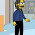## Friday, July 3, 2009

### A little Scheme code

Patrick Stein commented on the way that I defined my DTFT function:
```(define (dtft samples)
(lambda (omega)
(sum 0 (vector-length samples)
(lambda (n)
(* (vector-ref samples n)
(make-polar 1 (* omega n)))))))
```
Let me tweak it just a bit. The idea is that we have our original function which may or may not be continuous and we sample it at regular intervals.
```(define (dtft original sample-interval sample-count)
(lambda (omega)
(sum 0 sample-count
(lambda (n)
(* (original (* sample-interval n))
(make-polar 1 (* omega n)))))))
```
Now we need to turn our vector of samples into a function.
```;; Non robust.  Assumes N is a fixnum in the right range.
(define (vector->function sample-vector)
(lambda (n) (vector-ref sample-vector n)))
```
I take the original function and return a new function with a single parameter `omega` which is the transformed version. The original function only needs to be defined at the sample points in the range from `0` to `sample-count`. I'm treating my vector of samples as a function from `integer->real` (the samples are in fact integers, but they could be floats).

The function I return is a continuous function from `real->complex`. It is defined over all the reals, but since `omega` is an angle, the value computed is going to be repeated every time omega sweeps out an entire circle, so we should limit our interest to the range of 0 to 2π or -π to π.

It turns out to be somewhat computationally expensive to evaluate the transformed function. It isn't very expensive, but with eight thousand samples, we several tens of thousands of floating point operations. If we memoize the function, we can save a huge amount of time if we ever re-evaluate with the same omega. (And we will.)
```(define (memoize f)
(let ((table (make-eqv-hash-table))) ;; eqv works on floats
(lambda (arg)
(or (hash-table/get table arg #f)
(let ((value (f arg)))
(hash-table/put! table arg value)
value)))))

(define ym
(memoize
(dtft (vector->function *data*) 1 8192)))
```
The inverse transform is fairly simple:
```(define (inverse-dtft y domega)
(lambda (n)
(define (f omega)
(* (y omega)
(make-polar 1 (* omega (- n)))))

(/ (real-part (integrate f (- *pi*) *pi* domega))
(* *pi* 2))))
```
The integral should return a complex number where the real part cancels out to zero, but since the integration is a numeric approximation, I just lop off the imaginary part.

We need a definition of integrate. I got this simple trapezoid integration from Sussman and Abelson:
```(define (trapezoid f a b h)
(let* ((n (/ 1 h))
(dx (/ (- b a) n)))
(define (iter j sum)
(if (>= j n)
sum
(let ((x (+ a (* j dx))))
(iter (+ j 1) (+ sum (f x))))))
(* dx (iter 1 (+ (/ (f a) 2) (/ (f b) 2))))))

(define integrate trapezoid)
```
It converges slowly.
```(do ((i 1 (+ i 1))
(d-omega 1.0 (/ d-omega 2)))
((>= i 13))
(display i)
(display " ")
(display d-omega)
(display " ")
(display ((inverse-dtft ym d-omega) 250))
(newline))

1 1. -161.
2 .5 32215.
3 .25 16091.999999999949
4 .125 8074.
5 .0625 4074.000000000024
6 .03125 2048.0000000000246
7 .015625 1050.0000000000236
8 .0078125 571.9999999999932
9 .00390625 336.9999999999946
10 .001953125 235.99999999999997
11 .0009765625 184.9999999999997
12 .00048828125 160.99999999999937
13 .000244140625 153.00000000000196
```
But once I have it computed for one value of x, the others come fast. The actual value of the integral at this point is 150, but 153 is close enough for me.

1.You never did say what your hairy
function was measuring. Something
interesting?

-- Paul (who has rather less hair than once)

2.This comment has been removed by the author.

3.It is interesting, but I cannot yet comment on the source of the data.